Learning Objective

In this lesson we will learn how to write chemical equations in a way that reflects the Law of Conservation of Mass.

Learning Outcomes

By the end of this lesson you will be able to:

• Define the Law of Conservation of Mass.

• Explain how the Law of Conservation of Mass applies to chemical reactions.

• Write a balanced chemical equation.

BUY THE YEAR 9 CHEMISTRY WORKBOOK

## Conservation of Mass

• The Law of Conservation of Mass states that, during a physical or chemical change, matter is neither created nor destroyed.
• Therefore, for chemical reactions:
1. The total numbers of each type of atom are the same before and after a reaction has occurred.
2. The total mass of the products will be equal to the total mass of the reactants.
• In other words, although chemical reactions involve the formation of new substances, they don’t involve the formation of new atoms.
The only difference between reactants and products is how atoms are arranged, as a result of the breaking and forming of chemical bonds.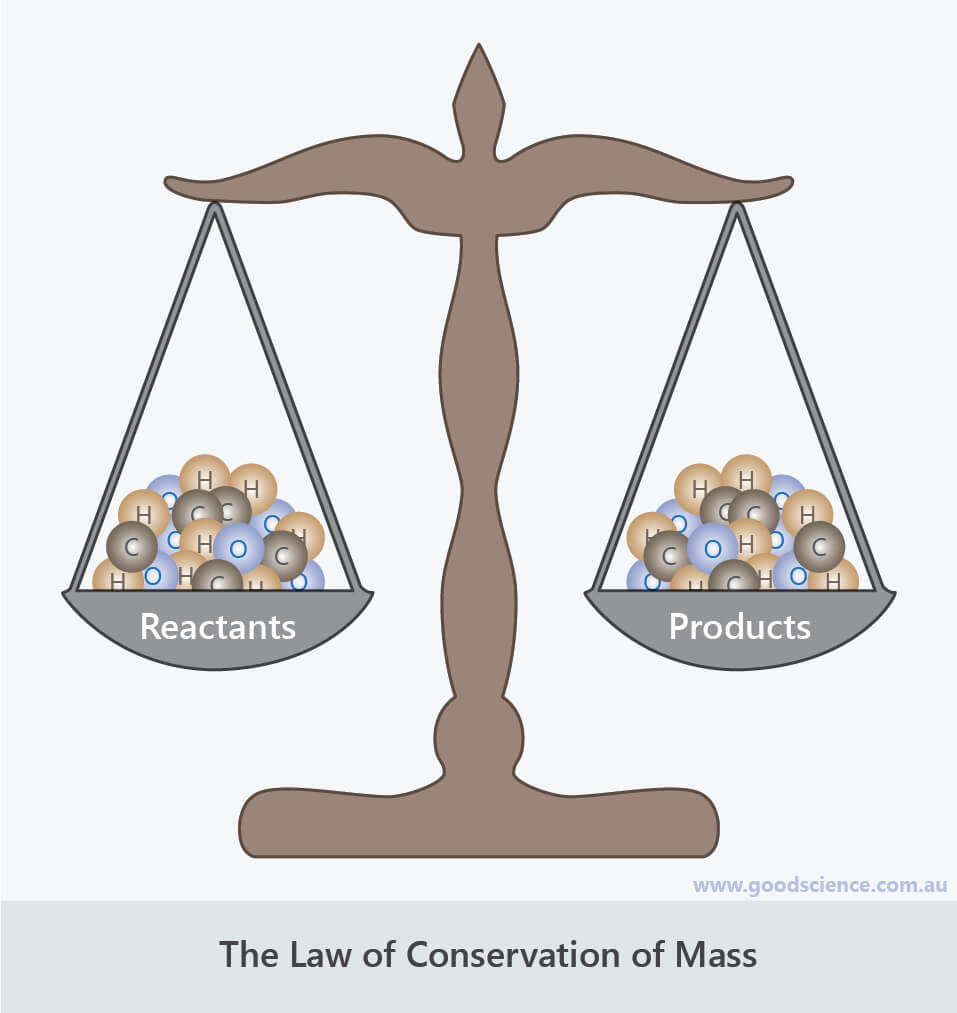For any chemical reaction, the mass of reactants is equal to the mass of products.

## Chemical Equations

• So far we have learned two ways of writing chemical equations to represent the reactants and products of a chemical reaction:
1. Word equations, which show the chemical names of all reactants and products.
2. Formula equations, which show the chemical formulas of all reactants and products.
• Often a formula equation doesn’t show equal numbers of each type of atom on both sides of the equation.
Consider the following equation for the reaction between nitrogen and hydrogen to form ammonia:The left side of the equation (the reactants) shows 2 nitrogen atoms whereas the right side of the equation (the product) shows 1 nitrogen atom.
Similarly, the left side of the equation shows 2 hydrogen atoms whereas the right side of the equation shows 3 hydrogen atoms.
• When a formula equation shows unequal numbers of atoms on either side of the equation, the equation is said to be unbalanced.
The equation is not demonstrating conservation of mass.
• To demonstrate conservation of mass by having equal numbers of atoms on either side of the equation, the equation needs to be balanced.

## Balancing Chemical Equations

• When first learning how to balance chemical equations, it can be helpful to draw diagrams of reactants and products.
For example, the above reaction between nitrogen and hydrogen can be illustrated by the following diagram: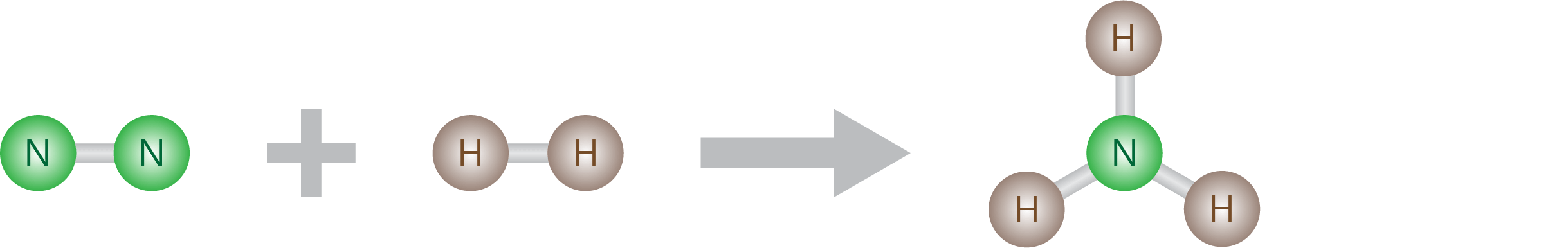• We can also create a tally to show the total number of each type of atom for both sides of the equation:

 Type of Atom Number of Atoms in Reactants Number of Atoms in Products N 2 1 H 2 3

• If a formula equation is unbalanced when it is first written, it means that the reactants and products do not exist in equal ratios.
For example, in the above reaction, one nitrogen molecule does not combine with one hydrogen molecule to form one ammonia molecule.
Therefore we need to determine the correct ratio for all reactants and products that will result in equal numbers of each type of atom on both sides of the equation.
• When balancing a chemical equation, deal with one type of atom at a time.
Let’s balance the above equation by first balancing the number of nitrogen atoms.
We will use diagrams and tallies to help us.
• The only way to make the number of atoms equal on both sides of an equation is to add more reactant or product molecules.
For example, in the above equation we can’t simply add 1 nitrogen atom to the products to balance the number of nitrogen atoms.
The only way we can increase the number of nitrogen atoms on the right side as to add another ammonia molecule: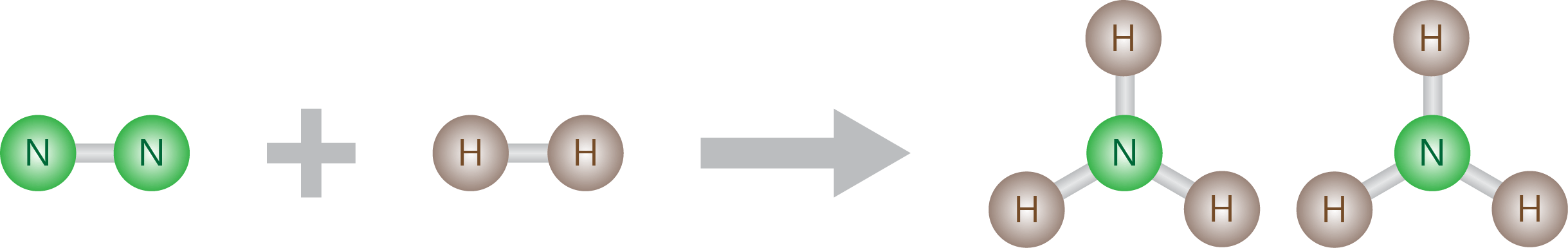• Now our diagram shows 2 nitrogen atoms on both sides of the equation.
Let’s update the tally:

 Type of Atom Number of Atoms in Reactants Number of Atoms in Products N 2 2 H 2 6

• Now we have balanced the number of nitrogen atoms, but the number of hydrogen atoms remains unbalanced. We have 2 hydrogen atoms on the left and 6 on the right.
The only way to increase the number of hydrogen atoms on the left is to add hydrogen molecules. To have 6 hydrogen atoms in total, we need 3 hydrogen molecules, so we need to add 2 more: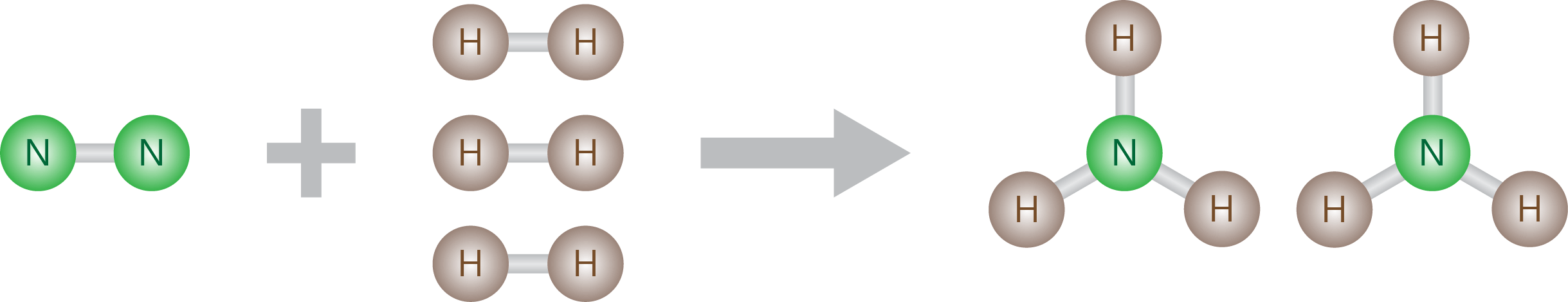• Now our diagram shows 6 hydrogen atoms on both sides of the equation.
Let’s update the tally:

 Type of Atom Number of Atoms in Reactants Number of Atoms in Products N 2 2 H 6 6

• Now we have balanced the number of nitrogen atoms and the number of hydrogen atoms.
The last step is to rewrite the formula equation so that it corresponds with our diagram.
We do this by adding numbers called coefficients in front of the chemical formulas of reactants and products that have more than one copy.
So we need to add a “3” in front of H2 to represent 3 hydrogen molecules, and a “2” in front of NH3 to represent 2 ammonia molecules.
We don’t add a “1” in front of N2 to represent 1 nitrogen molecule (just as we don’t have a subscript 1 next to the N in ammonia to represent 1 nitrogen atom).
Therefore our balance equation is:## Tips for Balancing Chemical Equations

• When first learning to balance chemical equations, use diagrams and tallies.
• Only adjust one type of atom at a time.
• Remember that coefficients change the tallies for all atoms in a substance, not just the atom you are trying to balance.
• If the equation contains elements, leave them until last when adjusting coefficients. This is because they can be adjusted without affecting the tallies of other atoms.
• You can only balance an equation by placing coefficients in front of substances. Never adjust the subscript numbers that are part of a chemical formula.
For example, you can’t change H2O to H2O2, it must be written as 2 H2O if you wish to double the number of oxygen atoms.
• Only place whole numbers (2, 3, 4 etc) in front of chemical formulas. If you find that a fraction is required to balance an equation, multiply all coefficients by the smallest number required to convert the fraction to a whole number.
• Polyatomic groups, such as NO3 and SO4, often stay together in reactions. If this is the case, consider them as one group in the tally, rather than as individual atoms – this will simplify the process.
• Check that the coefficients are the smallest possible numbers.
• For example,  4 H2 + 2 O2 4 H2O  can be simplified to  2 H2 + O2 2 H2O.

## Summary

• The Law of Conservation of Mass states that matter is neither created nor destroyed.
• To reflect the conservation of mass in a chemical reaction, chemical equations need to be balanced.
Equations are balanced by adding coefficients (numbers) in front of the chemical formulas of reactants and products.
• A balanced equation shows equal numbers of each type of atom on both sides of the equation.
It reflects the ratios of each reactant and product involved in the reaction.
• Steps for writing a balanced chemical equation:
1. Write the formula equation showing all reactants and products.
2. Tally up the total numbers of each type of atom for both sides of the equation.
3. Place coefficients in front of reactants and products until there are equal numbers of each type of atom on both sides of the equation.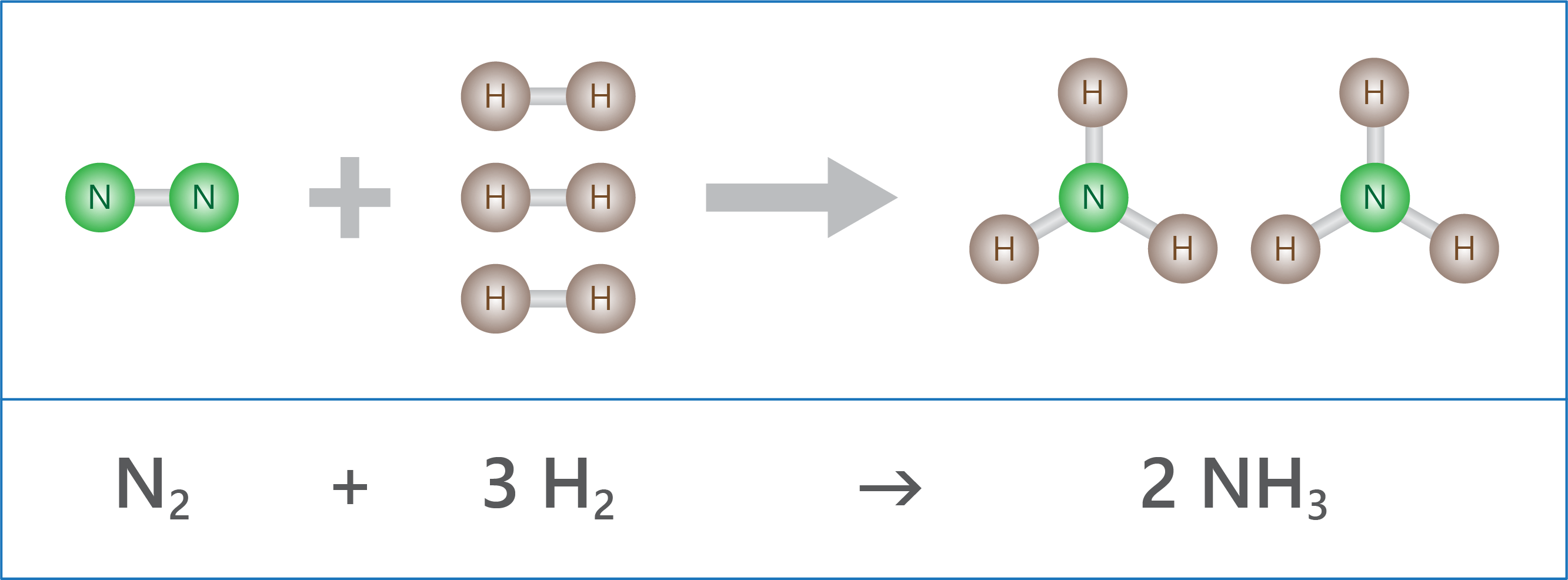BUY THE YEAR 9 CHEMISTRY WORKBOOK